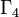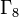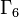# Element structure of groups of order 64

This article gives specific information, namely, element structure, about a family of groups, namely: groups of order 64.
View element structure of group families | View element structure of groups of a particular order |View other specific information about groups of order 64

## Conjugacy class sizes

FACTS TO CHECK AGAINST FOR CONJUGACY CLASS SIZES AND STRUCTURE:
Divisibility facts: size of conjugacy class divides order of group | size of conjugacy class divides index of center | size of conjugacy class equals index of centralizer
Bounding facts: size of conjugacy class is bounded by order of derived subgroup
Counting facts: number of conjugacy classes equals number of irreducible representations | class equation of a group

### Grouping by conjugacy class sizes

Here now is a grouping by conjugacy class sizes. Note that since number of conjugacy classes in group of prime power order is congruent to order of group modulo prime-square minus one, all the values for the number of conjugacy classes are congruent to 64 mod 3, and hence congruent to 1 mod 3.

Number of size 1 conjugacy classes Number of size 2 conjugacy classes Number of size 4 conjugacy classes Number of size 8 conjugacy classes Number of size 16 conjugacy classes Total number of conjugacy classes Total number of groups Nilpotency class(es) attained by these groups Hall-Senior family/families List of GAP IDs (second part)
64 0 0 0 0 64 11 1$\Gamma_1$, i.e., all the abelian groups of order 64 [SHOW MORE]
16 24 0 0 0 40 31 2$\Gamma_2$ [SHOW MORE]
8 12 8 0 0 28 60 2,3$\Gamma_3$ (class three) and$\Gamma_4$ (class two) [SHOW MORE]
4 30 0 0 0 34 7 2$\Gamma_5$ [SHOW MORE]
4 14 0 4 0 22 23 3, 4$\Gamma_8$ [SHOW MORE]
4 6 12 0 0 22 38 2,3$\Gamma_6$ and$\Gamma_7$ [SHOW MORE]

### Grouping by cumulative conjugacy class sizes (number of elements)

Number of elements in (size at most 1) conjugacy classes Number of elements in (size at most 2) conjugacy classes Number of elements in (size at most 4) conjugacy classes Number of elements in (size at most 8) conjugacy classes Number of elements in (size at most 16) conjugacy classes Total number of conjugacy classes Total number of groups Nilpotency class(es) attained by these groups Hall-Senior family/families List of GAP IDs (second part)
64 64 64 64 64 64 11 1$\Gamma_1$, i.e., all the abelian groups of order 64 [SHOW MORE]
16 64 64 64 64 40 31 2$\Gamma_2$ [SHOW MORE]
8 32 64 64 64 28 60 2,3$\Gamma_3$ (class three) and$\Gamma_4$ (class two) [SHOW MORE]
4 64 64 64 64 34 7 2$\Gamma_5$ [SHOW MORE]
4 32 32 64 64 22 23 3, 4$\Gamma_8$ [SHOW MORE]
4 16 64 64 64 24 38 2,3$\Gamma_6$ and$\Gamma_7$ [SHOW MORE]

Note that it is not true that the cumulative conjugacy class size statistics values divide the order of the group in all cases. There are a few counterexamples in the table above, as we can see values such as 12, 20, 28, and 40.$p^6$ is the smallest prime power where such examples exist. See also:

## 1-isomorphism

### Pairs where one of the groups is abelian

There are 29 pairs of groups that are 1-isomorphic with the property that one of them is abelian. Of these, some pairs share the abelian group part, as the table below shows. Of these, the only example of a group that is not of nilpotency class two is SmallGroup(64,25) (GAP ID: 25):

Here is a summary version:

Nature of 1-isomorphism Intermediate object Number of 1-isomorphisms between non-abelian and abelian group of this type Number of 1-isomorphisms between non-abelian and abelian group of this nature, not of any of the preceding types Note
linear halving generalization of Baer correspondence class two Lie ring 3 3 The examples are SmallGroup(64,57), direct product of SmallGroup(32,4) and Z2 (ID: (64,84)), and semidirect product of Z16 and Z4 of M-type (ID: (64,27)). See table below.
cocycle halving generalization of Baer correspondence class two Lie cring 17 14 See table below.
cocycle skew reversal generalization of Baer correspondence class two near-Lie cring 19 2 The two new examples are SmallGroup(64,17) and direct product of SmallGroup(32,2) and Z2.
linear halving generalization of Lazard correspondence class three Lie ring 4 0 Of the four examples, three arise via the linear halving generalization of Baer correspondence. The fourth, namely semidirect product of Z8 and Z8 of M-type (ID: (64,3)), alternatively arises via the cocycle halving generalization of Baer correspondence.
cocycle halving generalization of Lazard correspondence class three Lie cring 21 4 The new examples are semidirect product of Z16 and Z4 via fifth power map (ID: 28), SmallGroup(64,113), SmallGroup(64,114) and SmallGroup(64,210). See table below.

A total of 23 of the 29 1-isomorphisms are explained using the explanations here. Here is a long version:

Non-abelian member of pair GAP ID Abelian member of pair GAP ID The 1-isomorphism arises as a ... Description of the 1-isomorphism Best perspective 1 Best perspective 2 Alternative perspective
semidirect product of Z16 and Z4 of M-type 27 direct product of Z16 and Z4 26 linear halving generalization of Baer correspondence, the intermediate object being a class two Lie ring second cohomology group for trivial group action of direct product of Z4 and Z4 on Z4
SmallGroup(64,57) 57 direct product of Z4 and Z4 and Z4 55 linear halving generalization of Baer correspondence, the intermediate object being a class two Lie ring second cohomology group for trivial group action of direct product of Z4 and Z4 on Z4
direct product of SmallGroup(32,4) and Z2 84 direct product of Z8 and Z4 and Z2 83 linear halving generalization of Baer correspondence, the intermediate object being a class two Lie ring second cohomology group for trivial group action of direct product of Z4 and Z4 on Z4
semidirect product of Z8 and Z8 of M-type 3 direct product of Z8 and Z8 2 cocycle halving generalization of Baer correspondence, the intermediate object being a class two Lie cring; alternatively, the linear halving generalization of Lazard correspondence, the intermediate object being a class three Lie ring
M64 51 direct product of Z32 and Z2 50 cocycle halving generalization of Baer correspondence, the intermediate object being a class two Lie cring
direct product of M16 and Z4 85 direct product of Z8 and Z4 and Z2 83 cocycle halving generalization of Baer correspondence, the intermediate object being a class two Lie cring
central product of M16 and Z8 over common Z2 86 direct product of Z8 and Z4 and Z2 83 cocycle halving generalization of Baer correspondence, the intermediate object being a class two Lie cring
112 direct product of Z8 and Z4 and Z2 83 cocycle halving generalization of Baer correspondence, the intermediate object being a class two Lie cring
direct product of M32 and Z2 184 direct product of Z16 and V4 183 cocycle halving generalization of Baer correspondence, the intermediate object being a class two Lie cring
central product of D8 and Z16 185 direct product of Z16 and V4 183 cocycle halving generalization of Baer correspondence, the intermediate object being a class two Lie cring
direct product of SmallGroup(32,24) and Z2 195 direct product of Z4 and Z4 and V4 192 cocycle halving generalization of Baer correspondence, the intermediate object being a class two Lie cring
direct product of SmallGroup(16,13) and Z4 198 direct product of Z4 and Z4 and V4 192 cocycle halving generalization of Baer correspondence, the intermediate object being a class two Lie cring
direct product of M16 and V4 247 direct product of Z8 and E8 246 cocycle halving generalization of Baer correspondence, the intermediate object being a class two Lie cring
SmallGroup(64,248) 248 direct product of Z8 and E8 246 cocycle halving generalization of Baer correspondence, the intermediate object being a class two Lie cring
249 direct product of Z8 and E8 246 cocycle halving generalization of Baer correspondence, the intermediate object being a class two Lie cring
direct product of SmallGroup(16,13) and V4 263 direct product of E16 and Z4 260 cocycle halving generalization of Baer correspondence, the intermediate object being a class two Lie cring
266 direct product of E16 and Z4 260 cocycle halving generalization of Baer correspondence, the intermediate object being a class two Lie cring
SmallGroup(64,17) 17 direct product of Z8 and Z4 and Z2 83 cocycle skew reversal generalization of Baer correspondence, the intermediate object being a class two near-Lie cring
direct product of SmallGroup(32,2) and Z2 56 direct product of Z4 and Z4 and V4 192 cocycle skew reversal generalization of Baer correspondence, the intermediate object being a class two near-Lie cring
semidirect product of Z16 and Z4 via fifth power map 28 direct product of Z16 and Z4 26 cocycle halving generalization of Lazard correspondence, the intermediate object being a class three Lie cring
SmallGroup(64,113) 113 direct product of Z8 and Z4 and Z2 83 cocycle halving generalization of Lazard correspondence, the intermediate object being a class three Lie cring
SmallGroup(64,114) 114 direct product of Z8 and Z4 and Z2 83 cocycle halving generalization of Lazard correspondence, the intermediate object being a class three Lie cring
SmallGroup(64,210) 210 direct product of Z4 and Z4 and V4 192 cocycle halving generalization of Lazard correspondence, the intermediate object being a class three Lie cring
64 direct product of Z4 and Z4 and Z4 55  ?
82 direct product of Z4 and Z4 and Z4 55  ?
SmallGroup(64,25) 25 direct product of Z8 and Z4 and Z2 83  ?
61 direct product of Z4 and Z4 and V4 192  ?
77 direct product of Z4 and Z4 and V4 192  ?
direct product of SmallGroup(32,33) and Z2 209 direct product of Z4 and Z4 and V4 192

### Grouping by abelian member

Of the 11 abelian groups of order 64, 9 are 1-isomorphic to non-abelian groups. The only two that aren't are cyclic group:Z64, on account of the fact that finite group having the same order statistics as a cyclic group is cyclic, and elementary abelian group:E64, on account of the fact that exponent two implies abelian.

Abelian member GAP ID Total number of members (excluding abelian member) Other members GAP IDs (in order of listing) Hall-Senior symbols (in order of listing) Hall-Senior numbers (in order of listing)
direct product of Z8 and Z8 2 1 semidirect product of Z8 and Z8 of M-type 3
direct product of Z16 and Z4 26 2 semidirect product of Z16 and Z4 of M-type, semidirect product of Z16 and Z4 via fifth power map 27, 28
direct product of Z32 and Z2 50 1 M64 51
direct product of Z4 and Z4 and Z4 55 3 SmallGroup(64,57), SmallGroup(64,64), SmallGroup(64,82) 57, 64, 82
direct product of Z8 and Z4 and Z2 83 8 [SHOW MORE] 17, 25, 84, 85, 86, 112, 113, 114
direct product of Z16 and V4 183 2 direct product of M32 and Z2, central product of D8 and Z16 184, 185
direct product of Z4 and Z4 and V4 192 7 [SHOW MORE] 56, 61, 77, 195, 198, 209, 210
direct product of Z8 and E8 246 3 direct product of M16 and V4, SmallGroup(64,248), SmallGroup(64,249) 247, 248, 249
direct product of E16 and Z4 260 2 direct product of SmallGroup(16,13) and V4, SmallGroup(64,266) 263, 266
Total -- 29 -- -- -- --